# ISEE Upper Level Quantitative : How to add exponents

## Example Questions

### Example Question #21 : Exponential Operations

Two quantities are given - one in Column A and the other in Column B. Compare the quantities in the two columns.

Assume, in both columns, that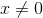.

Column A                       Column B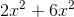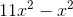The quantities in both columns are equal.

The quantity in Column B is greater.

The quantity in Column A is greater.

The relationship cannot be determined from the info given.

The quantity in Column B is greater.

Explanation:

When you are adding and subtracting terms with exponents, you combine like terms. Since both columns have expressions with the same exponent throughout, you are good to just look at the coefficients. Remember, a coefficient is the number in front of a variable. Therefore, Column A is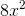since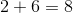. Column B is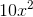since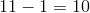. We can see that Column B is greater.

### Example Question #1 : How To Add Exponents

Add all of the perfect squares between 50 and 100 inclusive.

The correct answer is not among the other choices.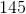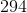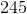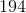Explanation:

The perfect squares between 50 and 100 inclusive are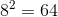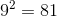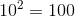Their sum is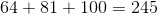### Example Question #1 : How To Add Exponents

Which is the greater quantity?

(A) The sum of the first ten perfect square integers

(B) The sum of the first five perfect cube integers

(B) is greater

It is impossible to determine which is greater from the information given

(A) and (B) are equal

(A) is greater

(A) is greater

Explanation:

The sum of the first ten perfect square integers: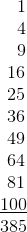The sum of the first five perfect cube integers: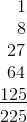(A) is greater.